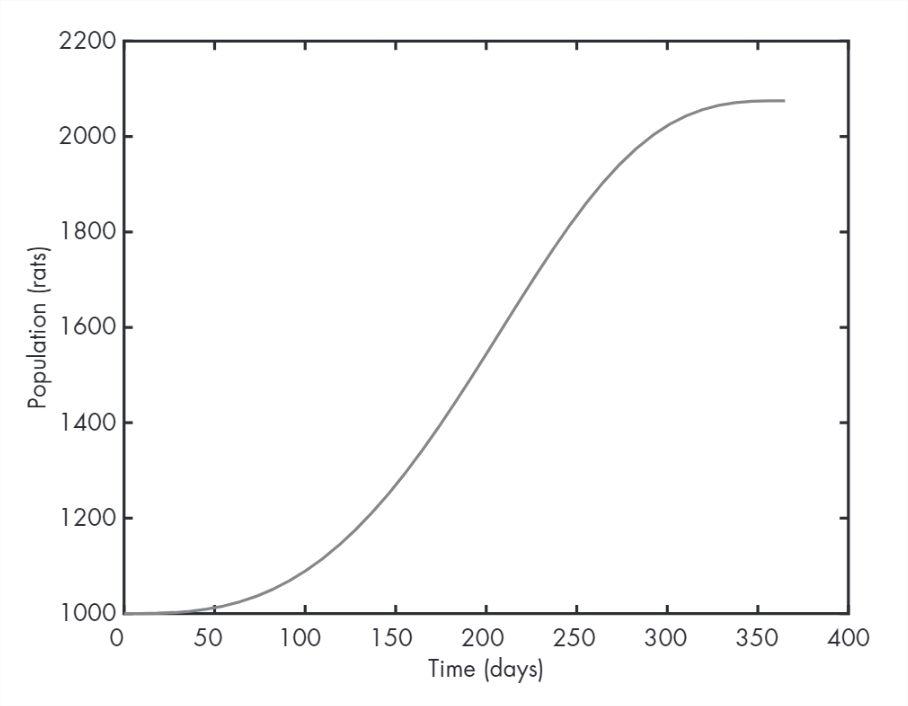# 19.6: Time Dependence

$$\newcommand{\vecs}{\overset { \rightharpoonup} {\mathbf{#1}} }$$ $$\newcommand{\vecd}{\overset{-\!-\!\rightharpoonup}{\vphantom{a}\smash {#1}}}$$$$\newcommand{\id}{\mathrm{id}}$$ $$\newcommand{\Span}{\mathrm{span}}$$ $$\newcommand{\kernel}{\mathrm{null}\,}$$ $$\newcommand{\range}{\mathrm{range}\,}$$ $$\newcommand{\RealPart}{\mathrm{Re}}$$ $$\newcommand{\ImaginaryPart}{\mathrm{Im}}$$ $$\newcommand{\Argument}{\mathrm{Arg}}$$ $$\newcommand{\norm}{\| #1 \|}$$ $$\newcommand{\inner}{\langle #1, #2 \rangle}$$ $$\newcommand{\Span}{\mathrm{span}}$$ $$\newcommand{\id}{\mathrm{id}}$$ $$\newcommand{\Span}{\mathrm{span}}$$ $$\newcommand{\kernel}{\mathrm{null}\,}$$ $$\newcommand{\range}{\mathrm{range}\,}$$ $$\newcommand{\RealPart}{\mathrm{Re}}$$ $$\newcommand{\ImaginaryPart}{\mathrm{Im}}$$ $$\newcommand{\Argument}{\mathrm{Arg}}$$ $$\newcommand{\norm}{\| #1 \|}$$ $$\newcommand{\inner}{\langle #1, #2 \rangle}$$ $$\newcommand{\Span}{\mathrm{span}}$$$$\newcommand{\AA}{\unicode[.8,0]{x212B}}$$

Looking at rate_func in the previous section, you might notice that it takes t as an input variable but doesn’t use it. That’s because the growth rate does not depend on time—bacteria don’t know what time it is.

But rats do. Or, at least, they know what season it is. Suppose that the growth rate for rats depends on the current population and the availability of food, which varies over the course of the year. The differential equation might be something like $\frac{dy}{dt}(t) = a y(t) \left(1 - \cos (\omega t) \right) \notag$ where $$t$$ is time in days and $$y(t)$$ is the population at time $$t$$. Because the growth rate depends on time, this differential equation is time dependent.

The variables $$a$$ and $$\omega$$ are parameters, which are values that quantify a physical aspect of the scenario. Parameters are often constants, but in some models they vary in time.

In this example, $$a$$ characterizes the reproductive rate per day, and $$\omega$$ is the frequency of a periodic function that describes the effect of varying food supply on reproduction.

We’ll use the values $$a = 0.002$$ and $$\omega = 2 \pi/365$$ (one cycle per year). The growth rate is lowest at $$t=0$$, on January 1, and highest at $$t=365/2$$, on June 30.

Now we can write a function that evaluates the growth rate:

function res = rate_func(t, y)
a = 0.002;
omega = 2*pi / 365;
res = a * y * (1 - cos(omega * t));
end

To test this function, I put it in a file called rats.m with a top-level function called rats:

function res = rats()
t = 365/2;
y = 1000;
res = rate_func(t, y);
end

The top-level function assumes, for purposes of testing, that there are 1000 rats at $$t=365/2$$ (June 30) and computes the growth rate under those conditions.

We can run the top-level function like this:

>> r = rats

r = 4

Under these conditions, the growth rate is 4 new rats per day.

Now that we’ve tested rate_func, we can use ode45 to solve the differential equation. Here’s how to call it from the top-level function in rats.m:

[T, Y] = ode45(@rate_func, [0, 365], 1000)
plot(T, Y)

The first argument is a function handle, again. The second argument is the interval we are interested in, a duration of one year, expressed in units of days. The third argument is the initial population, $$y(0) = 1000$$.

Figure 9.3 shows the results.Figure 9.3: Solutions to a simple differential equation by Euler’s method and ode45

The population grows slowly during the winter, quickly during the summer, and then slowly again in the fall.

To see the population at the end of the year, you can display the last element of Y:

Y(end)
2.0751e+03

That’s a little more than 2000 rats, so the population roughly doubles in one year.

The index here is end, which is a special word in MATLAB that means “the index of the last element.” You can use it in an expression, so Y(end - 1) is the second-to-last element of Y.

This page titled 19.6: Time Dependence is shared under a CC BY-NC-SA 4.0 license and was authored, remixed, and/or curated by Allen B. Downey (Green Tea Press) via source content that was edited to the style and standards of the LibreTexts platform; a detailed edit history is available upon request.